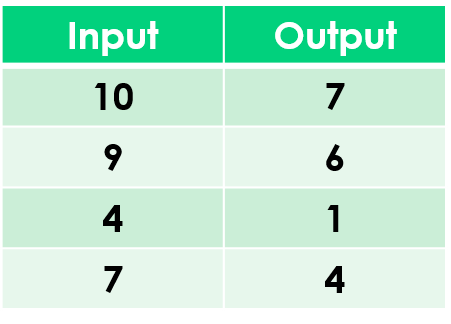1
visibility

Find the rule from the table below:• A

Subtract 3

• B

Subtract 1

The correct answer is "Subtract 3".

Please Note:
Subtraction is the operation that decreases a number by taking away from it.

Solution:
To find the correct rule, we consider the given table.

We observe that the given rule makes each number decrease. This implies subtraction.

We try subtracting 3 from each input number:

10 - 3 = 7
9 - 3 = 6
4 - 3 = 1
7 - 3 = 4

On subtracting 3 from the input numbers, we get the corresponding output numbers.

So, the rule is “subtract 3”.